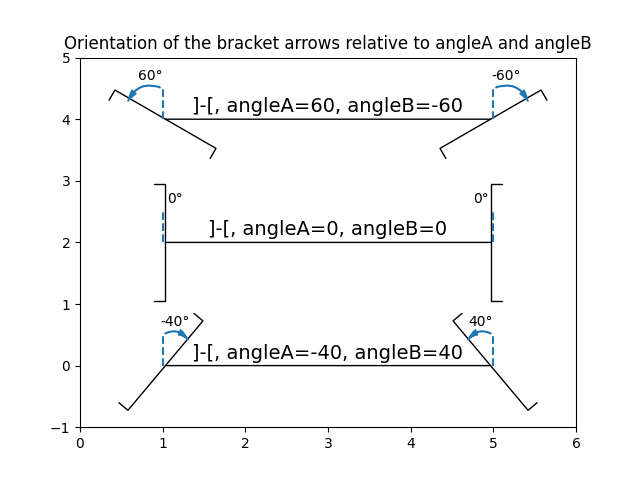# Angle annotations on bracket arrows#

This example shows how to add angle annotations to bracket arrow styles created using FancyArrowPatch. angleA and angleB are measured from a vertical line as positive (to the left) or negative (to the right). Blue FancyArrowPatch arrows indicate the directions of angleA and angleB from the vertical and axes text annotate the angle sizes.

import matplotlib.pyplot as plt
import numpy as np
from matplotlib.patches import FancyArrowPatch

def get_point_of_rotated_vertical(origin, line_length, degrees):
"""Return xy coordinates of the vertical line end rotated by degrees."""
return [origin + line_length * np.sin(rad),

fig, ax = plt.subplots()
ax.set(xlim=(0, 6), ylim=(-1, 5))
ax.set_title("Orientation of the bracket arrows relative to angleA and angleB")

style = ']-['
for i, angle in enumerate([-40, 0, 60]):
y = 2*i
arrow_centers = ((1, y), (5, y))
vlines = ((1, y + 0.5), (5, y + 0.5))
anglesAB = (angle, -angle)
bracketstyle = f"{style}, angleA={anglesAB}, angleB={anglesAB}"
bracket = FancyArrowPatch(*arrow_centers, arrowstyle=bracketstyle,
mutation_scale=42)
ax.text(3, y + 0.05, bracketstyle, ha="center", va="bottom", fontsize=14)
ax.vlines([line for line in vlines], [y, y], [line for line in vlines],
linestyles="--", color="C0")
# Get the top coordinates for the drawn patches at A and B
patch_tops = [get_point_of_rotated_vertical(center, 0.5, angle)
for center, angle in zip(arrow_centers, anglesAB)]
# Define the connection directions for the annotation arrows
connection_dirs = (1, -1) if angle > 0 else (-1, 1)
# Add arrows and annotation text
for vline, dir, patch_top, angle in zip(vlines, connection_dirs,
patch_tops, anglesAB):
arrowstyle=arrowstyle, color="C0")